# JavaScript 十大经典排序算法

### 排序算法说明

（1）排序的定义：对一序列对象根据某个关键字进行排序；

（3）对于评述算法优劣术语的说明

（4）排序算法图片总结(图片来源于网络):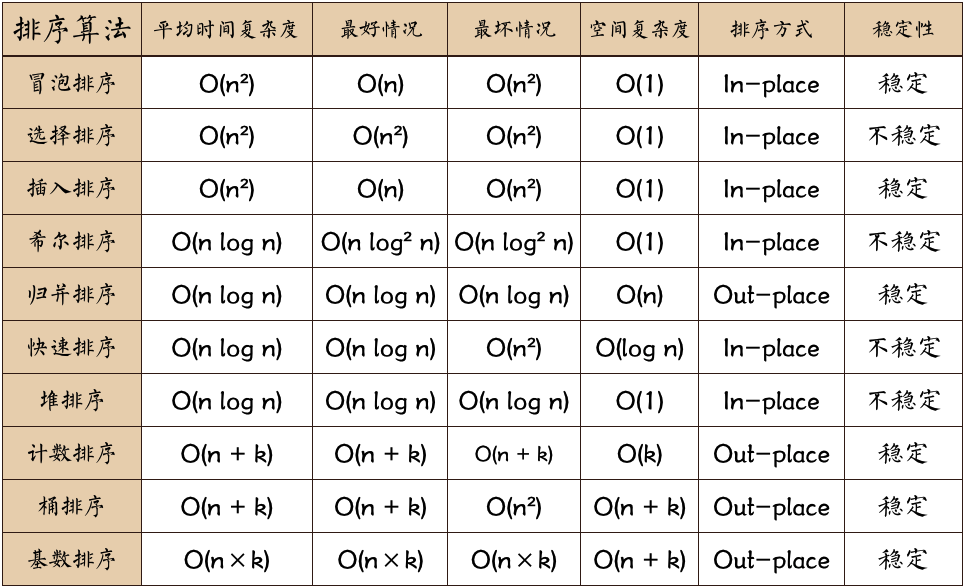n: 数据规模
k:“桶”的个数
In-place: 占用常数内存，不占用额外内存
Out-place: 占用额外内存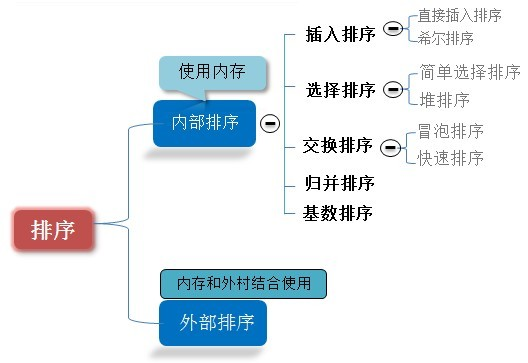### 1.冒泡排序（Bubble Sort）

#### (2)算法描述和实现

• <1>.比较相邻的元素。如果第一个比第二个大，就交换它们两个；
• <2>.对每一对相邻元素作同样的工作，从开始第一对到结尾的最后一对，这样在最后的元素应该会是最大的数；
• <3>.针对所有的元素重复以上的步骤，除了最后一个；
• <4>.重复步骤1~3，直到排序完成。

JavaScript代码实现：

function bubbleSort(arr) {
var len = arr.length;
for (var i = 0; i < len; i++) {
for (var j = 0; j < len - 1 - i; j++) {
if (arr[j] > arr[j+1]) {        //相邻元素两两对比
var temp = arr[j+1];        //元素交换
arr[j+1] = arr[j];
arr[j] = temp;
}
}
}
return arr;
}
var arr=[3,44,38,5,47,15,36,26,27,2,46,4,19,50,48];
console.log(bubbleSort(arr));//[2, 3, 4, 5, 15, 19, 26, 27, 36, 38, 44, 46, 47, 48, 50]


function bubbleSort2(arr) {
console.time('改进后冒泡排序耗时');
var i = arr.length-1;  //初始时,最后位置保持不变
while ( i> 0) {
var pos= 0; //每趟开始时,无记录交换
for (var j= 0; j< i; j++)
if (arr[j]> arr[j+1]) {
pos= j; //记录交换的位置
var tmp = arr[j]; arr[j]=arr[j+1];arr[j+1]=tmp;
}
i= pos; //为下一趟排序作准备
}
console.timeEnd('改进后冒泡排序耗时');
return arr;
}
var arr=[3,44,38,5,47,15,36,26,27,2,46,4,19,50,48];
console.log(bubbleSort2(arr));//[2, 3, 4, 5, 15, 19, 26, 27, 36, 38, 44, 46, 47, 48, 50]

function bubbleSort3(arr3) {
var low = 0;
var high= arr.length-1; //设置变量的初始值
var tmp,j;
console.time('2.改进后冒泡排序耗时');
while (low < high) {
for (j= low; j< high; ++j) //正向冒泡,找到最大者
if (arr[j]> arr[j+1]) {
tmp = arr[j]; arr[j]=arr[j+1];arr[j+1]=tmp;
}
--high;                 //修改high值, 前移一位
for (j=high; j>low; --j) //反向冒泡,找到最小者
if (arr[j]<arr[j-1]) {
tmp = arr[j]; arr[j]=arr[j-1];arr[j-1]=tmp;
}
++low;                  //修改low值,后移一位
}
console.timeEnd('2.改进后冒泡排序耗时');
return arr3;
}
var arr=[3,44,38,5,47,15,36,26,27,2,46,4,19,50,48];
console.log(bubbleSort3(arr));//[2, 3, 4, 5, 15, 19, 26, 27, 36, 38, 44, 46, 47, 48, 50]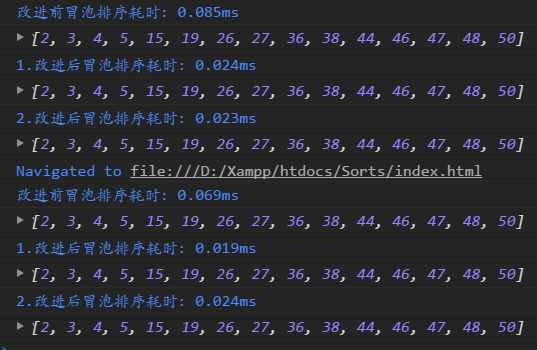(3)算法分析

• 最佳情况：T(n) = O(n)

• 最差情况：T(n) = O(n2)

• 平均情况：T(n) = O(n2)

### 2.选择排序（Selection Sort）

#### (2)算法描述和实现

n个记录的直接选择排序可经过n-1趟直接选择排序得到有序结果。具体算法描述如下：

• <1>.初始状态：无序区为R[1..n]，有序区为空；
• <2>.第i趟排序(i=1,2,3...n-1)开始时，当前有序区和无序区分别为R[1..i-1]和R(i..n）。该趟排序从当前无序区中-选出关键字最小的记录 R[k]，将它与无序区的第1个记录R交换，使R[1..i]和R[i+1..n)分别变为记录个数增加1个的新有序区和记录个数减少1个的新无序区；
• <3>.n-1趟结束，数组有序化了。

Javascript代码实现:

function selectionSort(arr) {
var len = arr.length;
var minIndex, temp;
console.time('选择排序耗时');
for (var i = 0; i < len - 1; i++) {
minIndex = i;
for (var j = i + 1; j < len; j++) {
if (arr[j] < arr[minIndex]) {     //寻找最小的数
minIndex = j;                 //将最小数的索引保存
}
}
temp = arr[i];
arr[i] = arr[minIndex];
arr[minIndex] = temp;
}
console.timeEnd('选择排序耗时');
return arr;
}
var arr=[3,44,38,5,47,15,36,26,27,2,46,4,19,50,48];
console.log(selectionSort(arr));//[2, 3, 4, 5, 15, 19, 26, 27, 36, 38, 44, 46, 47, 48, 50]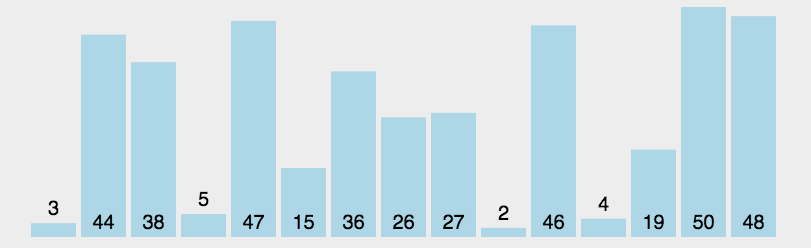#### (3)算法分析

• 最佳情况：T(n) = O(n2)
• 最差情况：T(n) = O(n2)
• 平均情况：T(n) = O(n2)

## 3.插入排序（Insertion Sort）

#### (2)算法描述和实现

• <1>.从第一个元素开始，该元素可以认为已经被排序；
• <2>.取出下一个元素，在已经排序的元素序列中从后向前扫描；
• <3>.如果该元素（已排序）大于新元素，将该元素移到下一位置；
• <4>.重复步骤3，直到找到已排序的元素小于或者等于新元素的位置；
• <5>.将新元素插入到该位置后；
• <6>.重复步骤2~5。

Javascript代码实现:

function insertionSort(array) {
if (Object.prototype.toString.call(array).slice(8, -1) === 'Array') {
console.time('插入排序耗时：');
for (var i = 1; i < array.length; i++) {
var key = array[i];
var j = i - 1;
while (j >= 0 && array[j] > key) {
array[j + 1] = array[j];
j--;
}
array[j + 1] = key;
}
console.timeEnd('插入排序耗时：');
return array;
} else {
return 'array is not an Array!';
}
}


function binaryInsertionSort(array) {
if (Object.prototype.toString.call(array).slice(8, -1) === 'Array') {
console.time('二分插入排序耗时：');

for (var i = 1; i < array.length; i++) {
var key = array[i], left = 0, right = i - 1;
while (left <= right) {
var middle = parseInt((left + right) / 2);
if (key < array[middle]) {
right = middle - 1;
} else {
left = middle + 1;
}
}
for (var j = i - 1; j >= left; j--) {
array[j + 1] = array[j];
}
array[left] = key;
}
console.timeEnd('二分插入排序耗时：');

return array;
} else {
return 'array is not an Array!';
}
}
var arr=[3,44,38,5,47,15,36,26,27,2,46,4,19,50,48];
console.log(binaryInsertionSort(arr));//[2, 3, 4, 5, 15, 19, 26, 27, 36, 38, 44, 46, 47, 48, 50]#### (3)算法分析

• 最佳情况：输入数组按升序排列。T(n) = O(n)
• 最坏情况：输入数组按降序排列。T(n) = O(n2)
• 平均情况：T(n) = O(n2)

### 4.希尔排序（Shell Sort）

1959年Shell发明；

#### (2)算法描述和实现

• <1>. 选择一个增量序列t1，t2，…，tk，其中ti>tj，tk=1；
• <2>.按增量序列个数k，对序列进行k 趟排序；
• <3>.每趟排序，根据对应的增量ti，将待排序列分割成若干长度为m 的子序列，分别对各子表进行直接插入排序。仅增量因子为1 时，整个序列作为一个表来处理，表长度即为整个序列的长度。

Javascript代码实现：

function shellSort(arr) {
var len = arr.length,
temp,
gap = 1;
console.time('希尔排序耗时:');
while(gap < len/5) {          //动态定义间隔序列
gap =gap*5+1;
}
for (gap; gap > 0; gap = Math.floor(gap/5)) {
for (var i = gap; i < len; i++) {
temp = arr[i];
for (var j = i-gap; j >= 0 && arr[j] > temp; j-=gap) {
arr[j+gap] = arr[j];
}
arr[j+gap] = temp;
}
}
console.timeEnd('希尔排序耗时:');
return arr;
}
var arr=[3,44,38,5,47,15,36,26,27,2,46,4,19,50,48];
console.log(shellSort(arr));//[2, 3, 4, 5, 15, 19, 26, 27, 36, 38, 44, 46, 47, 48, 50]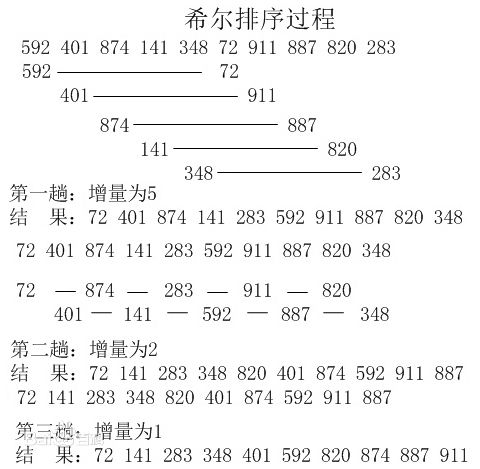#### （3）算法分析

• 最佳情况：T(n) = O(nlog2 n)
• 最坏情况：T(n) = O(nlog2 n)
• 平均情况：T(n) =O(nlog n)

### 5.归并排序（Merge Sort）

#### (1)算法简介

归并排序是建立在归并操作上的一种有效的排序算法。该算法是采用分治法（Divide and Conquer）的一个非常典型的应用。归并排序是一种稳定的排序方法。将已有序的子序列合并，得到完全有序的序列；即先使每个子序列有序，再使子序列段间有序。若将两个有序表合并成一个有序表，称为2-路归并。

#### (2)算法描述和实现

• <1>.把长度为n的输入序列分成两个长度为n/2的子序列；
• <2>.对这两个子序列分别采用归并排序；
• <3>.将两个排序好的子序列合并成一个最终的排序序列。

Javscript代码实现:

function mergeSort(arr) {  //采用自上而下的递归方法
var len = arr.length;
if(len < 2) {
return arr;
}
var middle = Math.floor(len / 2),
left = arr.slice(0, middle),
right = arr.slice(middle);
return merge(mergeSort(left), mergeSort(right));
}

function merge(left, right)
{
var result = [];
console.time('归并排序耗时');
while (left.length && right.length) {
if (left <= right) {
result.push(left.shift());
} else {
result.push(right.shift());
}
}

while (left.length)
result.push(left.shift());

while (right.length)
result.push(right.shift());
console.timeEnd('归并排序耗时');
return result;
}
var arr=[3,44,38,5,47,15,36,26,27,2,46,4,19,50,48];
console.log(mergeSort(arr));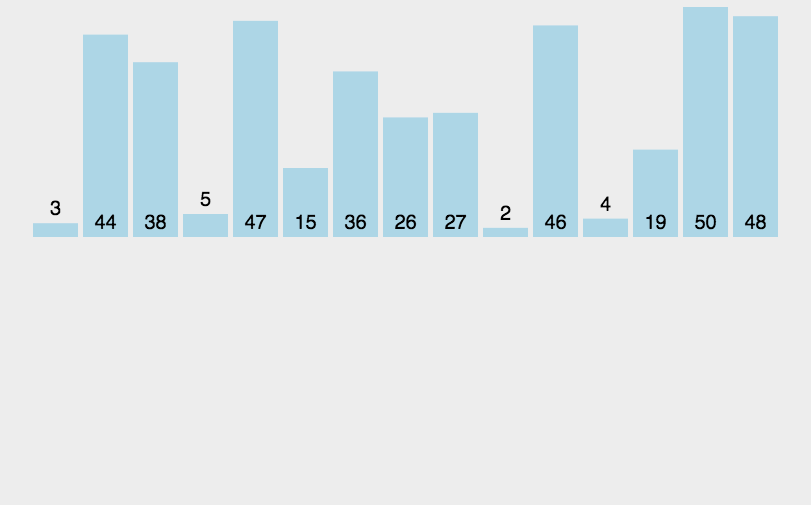#### (3)算法分析

• 最佳情况：T(n) = O(n)
• 最差情况：T(n) = O(nlogn)
• 平均情况：T(n) = O(nlogn)

### 6.快速排序（Quick Sort）

#### (2)算法描述和实现

• <1>.从数列中挑出一个元素，称为 "基准"（pivot）；
• <2>.重新排序数列，所有元素比基准值小的摆放在基准前面，所有元素比基准值大的摆在基准的后面（相同的数可以到任一边）。在这个分区退出之后，该基准就处于数列的中间位置。这个称为分区（partition）操作；
• <3>.递归地（recursive）把小于基准值元素的子数列和大于基准值元素的子数列排序。

Javascript代码实现：

/*方法说明：快速排序
@param  array 待排序数组*/
//方法一
function quickSort(array, left, right) {
console.time('1.快速排序耗时');
if (Object.prototype.toString.call(array).slice(8, -1) === 'Array' && typeof left === 'number' && typeof right === 'number') {
if (left < right) {
var x = array[right], i = left - 1, temp;
for (var j = left; j <= right; j++) {
if (array[j] <= x) {
i++;
temp = array[i];
array[i] = array[j];
array[j] = temp;
}
}
quickSort(array, left, i - 1);
quickSort(array, i + 1, right);
}
console.timeEnd('1.快速排序耗时');
return array;
} else {
return 'array is not an Array or left or right is not a number!';
}
}

//方法二
var quickSort2 = function(arr) {
console.time('2.快速排序耗时');
if (arr.length <= 1) { return arr; }
var pivotIndex = Math.floor(arr.length / 2);
var pivot = arr.splice(pivotIndex, 1);
var left = [];
var right = [];
for (var i = 0; i < arr.length; i++){
if (arr[i] < pivot) {
left.push(arr[i]);
} else {
right.push(arr[i]);
}
}
console.timeEnd('2.快速排序耗时');
return quickSort2(left).concat([pivot], quickSort2(right));
};

var arr=[3,44,38,5,47,15,36,26,27,2,46,4,19,50,48];
console.log(quickSort(arr,0,arr.length-1));//[2, 3, 4, 5, 15, 19, 26, 27, 36, 38, 44, 46, 47, 48, 50]
console.log(quickSort2(arr));//[2, 3, 4, 5, 15, 19, 26, 27, 36, 38, 44, 46, 47, 48, 50]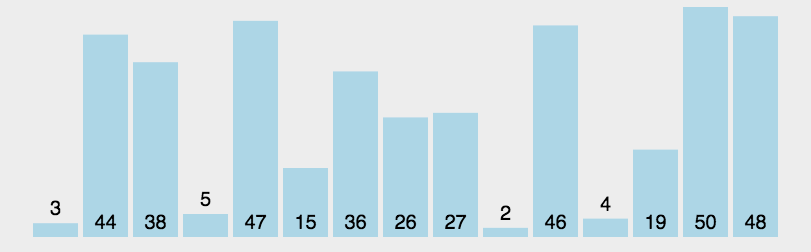#### (3)算法分析

• 最佳情况：T(n) = O(nlogn)
• 最差情况：T(n) = O(n2)
• 平均情况：T(n) = O(nlogn)

### 7.堆排序（Heap Sort）

#### (2)算法描述和实现

• <1>.将初始待排序关键字序列(R1,R2....Rn)构建成大顶堆，此堆为初始的无序区；
• <2>.将堆顶元素R与最后一个元素R[n]交换，此时得到新的无序区(R1,R2,......Rn-1)和新的有序区(Rn),且满足R[1,2...n-1]<=R[n]；
• <3>.由于交换后新的堆顶R可能违反堆的性质，因此需要对当前无序区(R1,R2,......Rn-1)调整为新堆，然后再次将R与无序区最后一个元素交换，得到新的无序区(R1,R2....Rn-2)和新的有序区(Rn-1,Rn)。不断重复此过程直到有序区的元素个数为n-1，则整个排序过程完成。

Javascript代码实现：

/*方法说明：堆排序
@param  array 待排序数组*/
function heapSort(array) {
console.time('堆排序耗时');
if (Object.prototype.toString.call(array).slice(8, -1) === 'Array') {
//建堆
var heapSize = array.length, temp;
for (var i = Math.floor(heapSize / 2) - 1; i >= 0; i--) {
heapify(array, i, heapSize);
}

//堆排序
for (var j = heapSize - 1; j >= 1; j--) {
temp = array;
array = array[j];
array[j] = temp;
heapify(array, 0, --heapSize);
}
console.timeEnd('堆排序耗时');
return array;
} else {
return 'array is not an Array!';
}
}
/*方法说明：维护堆的性质
@param  arr 数组
@param  x   数组下标
@param  len 堆大小*/
function heapify(arr, x, len) {
if (Object.prototype.toString.call(arr).slice(8, -1) === 'Array' && typeof x === 'number') {
var l = 2 * x + 1, r = 2 * x + 2, largest = x, temp;
if (l < len && arr[l] > arr[largest]) {
largest = l;
}
if (r < len && arr[r] > arr[largest]) {
largest = r;
}
if (largest != x) {
temp = arr[x];
arr[x] = arr[largest];
arr[largest] = temp;
heapify(arr, largest, len);
}
} else {
return 'arr is not an Array or x is not a number!';
}
}
var arr=[91,60,96,13,35,65,46,65,10,30,20,31,77,81,22];
console.log(heapSort(arr));//[10, 13, 20, 22, 30, 31, 35, 46, 60, 65, 65, 77, 81, 91, 96]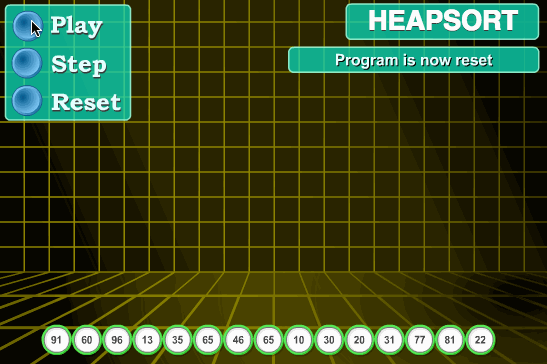#### (3)算法分析

• 最佳情况：T(n) = O(nlogn)
• 最差情况：T(n) = O(nlogn)
• 平均情况：T(n) = O(nlogn)

### 8.计数排序（Counting Sort）

#### (2)算法描述和实现

• <1>. 找出待排序的数组中最大和最小的元素；
• <2>. 统计数组中每个值为i的元素出现的次数，存入数组C的第i项；
• <3>. 对所有的计数累加（从C中的第一个元素开始，每一项和前一项相加）；
• <4>. 反向填充目标数组：将每个元素i放在新数组的第C(i)项，每放一个元素就将C(i)减去1。

Javascript代码实现：

function countingSort(array) {
var len = array.length,
B = [],
C = [],
min = max = array;
console.time('计数排序耗时');
for (var i = 0; i < len; i++) {
min = min <= array[i] ? min : array[i];
max = max >= array[i] ? max : array[i];
C[array[i]] = C[array[i]] ? C[array[i]] + 1 : 1;
}
for (var j = min; j < max; j++) {
C[j + 1] = (C[j + 1] || 0) + (C[j] || 0);
}
for (var k = len - 1; k >= 0; k--) {
B[C[array[k]] - 1] = array[k];
C[array[k]]--;
}
console.timeEnd('计数排序耗时');
return B;
}
var arr = [2, 2, 3, 8, 7, 1, 2, 2, 2, 7, 3, 9, 8, 2, 1, 4, 2, 4, 6, 9, 2];
console.log(countingSort(arr)); //[1, 1, 2, 2, 2, 2, 2, 2, 2, 2, 3, 3, 4, 4, 6, 7, 7, 8, 8, 9, 9]


JavaScript动图演示：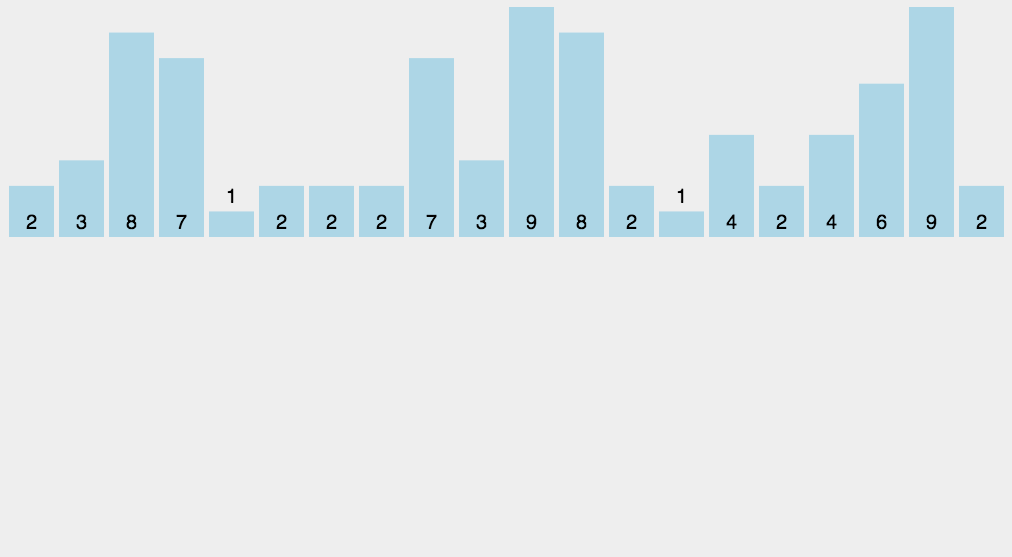#### (3)算法分析

• 最佳情况：T(n) = O(n+k)
• 最差情况：T(n) = O(n+k)
• 平均情况：T(n) = O(n+k)

### 9.桶排序（Bucket Sort）

#### (2)算法描述和实现

• <1>.设置一个定量的数组当作空桶；
• <2>.遍历输入数据，并且把数据一个一个放到对应的桶里去；
• <3>.对每个不是空的桶进行排序；
• <4>.从不是空的桶里把排好序的数据拼接起来。

Javascript代码实现:

/*方法说明：桶排序
@param  array 数组
@param  num   桶的数量*/
function bucketSort(array, num) {
if (array.length <= 1) {
return array;
}
var len = array.length, buckets = [], result = [], min = max = array, regex = '/^[1-9]+[0-9]*\$/', space, n = 0;
num = num || ((num > 1 && regex.test(num)) ? num : 10);
console.time('桶排序耗时');
for (var i = 1; i < len; i++) {
min = min <= array[i] ? min : array[i];
max = max >= array[i] ? max : array[i];
}
space = (max - min + 1) / num;
for (var j = 0; j < len; j++) {
var index = Math.floor((array[j] - min) / space);
if (buckets[index]) {   //  非空桶，插入排序
var k = buckets[index].length - 1;
while (k >= 0 && buckets[index][k] > array[j]) {
buckets[index][k + 1] = buckets[index][k];
k--;
}
buckets[index][k + 1] = array[j];
} else {    //空桶，初始化
buckets[index] = [];
buckets[index].push(array[j]);
}
}
while (n < num) {
result = result.concat(buckets[n]);
n++;
}
console.timeEnd('桶排序耗时');
return result;
}
var arr=[3,44,38,5,47,15,36,26,27,2,46,4,19,50,48];
console.log(bucketSort(arr,4));//[2, 3, 4, 5, 15, 19, 26, 27, 36, 38, 44, 46, 47, 48, 50]#### (3)算法分析

桶排序最好情况下使用线性时间O(n)，桶排序的时间复杂度，取决与对各个桶之间数据进行排序的时间复杂度，因为其它部分的时间复杂度都为O(n)。很显然，桶划分的越小，各个桶之间的数据越少，排序所用的时间也会越少。但相应的空间消耗就会增大。

• 最佳情况：T(n) = O(n+k)
• 最差情况：T(n) = O(n+k)
• 平均情况：T(n) = O(n2)

#### (2)算法描述和实现

• <1>.取得数组中的最大数，并取得位数；

Javascript代码实现：

/**
* 基数排序适用于：
*  (1)数据范围较小，建议在小于1000
*  (2)每个数值都要大于等于0
* @author xiazdong
* @param  arr 待排序数组
* @param  maxDigit 最大位数
*/

function radixSort(arr, maxDigit) {
var mod = 10;
var dev = 1;
var counter = [];
console.time('基数排序耗时');
for (var i = 0; i < maxDigit; i++, dev *= 10, mod *= 10) {
for(var j = 0; j < arr.length; j++) {
var bucket = parseInt((arr[j] % mod) / dev);
if(counter[bucket]== null) {
counter[bucket] = [];
}
counter[bucket].push(arr[j]);
}
var pos = 0;
for(var j = 0; j < counter.length; j++) {
var value = null;
if(counter[j]!=null) {
while ((value = counter[j].shift()) != null) {
arr[pos++] = value;
}
}
}
}
console.timeEnd('基数排序耗时');
return arr;
}
var arr = [3, 44, 38, 5, 47, 15, 36, 26, 27, 2, 46, 4, 19, 50, 48];
console.log(radixSort(arr,2)); //[2, 3, 4, 5, 15, 19, 26, 27, 36, 38, 44, 46, 47, 48, 50]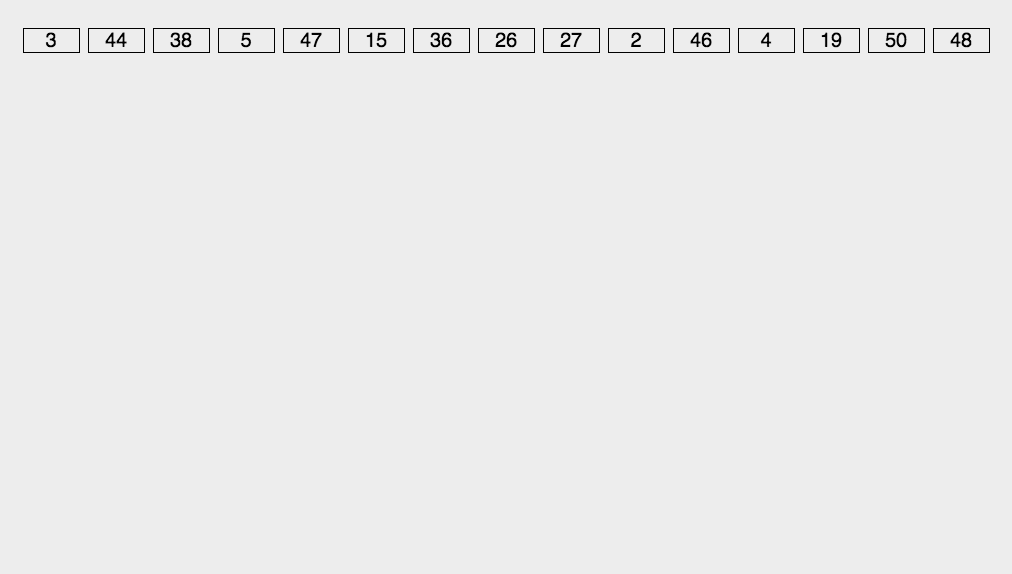#### (3)算法分析

• 最佳情况：T(n) = O(n * k)
• 最差情况：T(n) = O(n * k)
• 平均情况：T(n) = O(n * k)

• MSD 从高位开始进行排序
• LSD 从低位开始进行排序

1. 基数排序：根据键值的每位数字来分配桶
2. 计数排序：每个桶只存储单一键值
3. 桶排序：每个桶存储一定范围的数值